# A quick introduction to rarefaction analisys using Rarefy

#### 2021-03-11

#Required packages
require(Rarefy)
require(ade4)
require(adiv)
require(ape)
require(vegan)
require(phyloregion)
require(raster)

## Introduction

Rarefy is an R package including a set of new functions able to cope with any diversity metric and to calculate expected values of a given taxonomic, functional or phylogenetic index for a reduced sampling size under a spatially-constrained and distance-based ordering of the sampling units. Rarefy and the functions therein represent an ultimate solution for ecologists to rarefy any diversity metric by taking into account the contribution of any distance-based ordination of sampling units, providing more ecological meaningful (unbiased) estimates of the expected diversities.

This vignette aims at describing some applications of the Rarefy package helping the user to calculate different types of spatially and non-spatially explicit rarefaction curves. The vignette is organized in the following sections, exploring different package features and applications:

1. Diversity indices and dataset available in Rarefy;
2. Spatially-explicit rarefaction;
3. Rarefaction of alpha diversity indices;
4. Rarefaction of beta diversity;
5. Phylogenetic spatially-explicit rarefaction;
6. Null models in rarefaction practice.

## 1. Diversity indices and dataset available in Rarefy

Rarefy offers the possibility to calculate a large set of diversity indices and new metrics will be implemented in future packages updates. In the table below, for functions implemented in Rarefy, the set of indices available are detailed.

Functions Metrics Formula Description
$$\textbf{rare_alpha}$$ HCDT entropy (HCDT) $HCDT=\frac{\left(1-\sum_{i=1}^{n}p_i^q\right)}{\left(q-1\right)}$ HCDT entropy (Harvda & Charvat 1967; Daròczy 1970; Tsallis 1988) is a generalization of the standard coefficient of entropy. $$q$$ is the parameter that regulates the sensitivity to species abundance and $$p_i$$ is the relative abundance of species $$i$$. For $$q=0$$, the index is equal to species richness minus one, for $$q$$ tending to 1, it is equivalent to Shannon entropy and for $$q=2$$ it is equivalent to the Gini-Simpson index.
Hill numbers ($$^{q}D$$) $^{q}\textrm{D}=\left( \sum_{i=1}^{S}p_i^q\right )^{1/(1-q)}$ Hill numbers (Hill 1973) is a class of measures that obeys to the replication principle and integrates species richness and species abundances. The parameter $$q$$, called 'order', regulates the sensitivity of the index to the species abundance: with $$q=0$$ the value of the index corresponds to the species richness, with $$q$$ tending to 1 the measure tends to the exponential of Shannon index, and with $$q=2$$ it corresponds to the inverse of Simpson index. $$p_i$$ is the relative abundance of species $$i$$.
$$\textbf{rare_beta}$$ Bray-Curtis dissimilarity ($$\beta_{bray}$$) $\beta_{bray}=\frac{\sum_{i}(x_i-x_j)}{\sum_{i}x_i+x_j}$ Bray-Curtis dissimilarity (Bray & Curtis 1957) is a pairwise measure of similarity between plots weighted by the abundances of the species. The accumulation curve is calculated with the mean of pairwise dissimilarities among $$N$$ plots. $$x_i$$ and $$x_j$$ are the abundances of the species $$x$$ in the plots $$i$$ and $$j$$.
Cody index ($$\beta_{c}$$) $\beta_c=\frac{\left[g\left(H\right)+l\left(H\right)\right]}{2}$ Cody index (Cody 1975) is defined as the rate at which species are being replaced in censuses at each point on the habitat gradient and is fixed for samples arranged along gradients of environmental change. $$g(H)$$ is the number of species gained along the habitat gradient $$H$$ and $$l(H)$$ is the number of species lost.
Jaccard similarity coefficient ($$\beta_{j}$$) $\beta_j=\frac{a}{\left(\alpha_1+\alpha_2-a\right)}$ Jaccard dissimilarity coefficient (Jaccard 1901, 1912) is a pairwise measure of dissimilarity between plots. The accumulation curve is calculated with the mean of pairwise dissimilarities among $$N$$ plots. $$a$$ is the number of species in common between two plots, and $$\alpha_1$$ and $$\alpha_2$$ are the values of alpha diversity (species richness) of the 2 plots compared.
Whittaker's species turnover ($$\beta_{w}$$) $\beta_w=\frac{\gamma}{\alpha}-1$ Whittaker's species turnover (Whittaker 1960, 1972) calculates how many times there is a change in species composition among the plots.$$\gamma$$ is the species richness over all plots compared and $$\alpha$$ the average species richness within a single plot
$$\textbf{rare_phylo/ser_phylo}$$ Barker's weighted phylogenetic diversity (PDw) $PDw=B\times\frac{\sum_{i}^{B}{L_iA_i}}{\sum_{i}^{B}A_i}$ Barker's weighted phylogenetic diversity ($$PD_w$$) (Barker 2002) is the abundance weighted Faith's $$PD$$: the number of branches is multiplied by the weighted mean branch length, with weights equal to the average abundance of species sharing that branch. $$L_i$$ is the branch length of the branch $$i$$, and $$A_i$$ is the average abundance of the species sharing the branch $$i$$. $$B$$ is the number of branches in the tree.
Faith’s phylogenetic diversity (PD) $PD=\sum_{i\in B} L_i$ Faith’s phylogenetic diversity ($$PD$$) (Faith 1992), is defined as the sum of branch lengths in a phylogenetic tree for the assemblage of species. $$L_i$$ is the branch length of the branch $$i$$ and $$B$$ is the number of branches in the tree.
Feature diversity indexes (ƒdiv) $^qƒdiv_{Hill}=\left [\sum_{i \in B} L_{i}(p_{i})^{q}]\right ]^{\frac{1}{1-q}}$ $^qƒdiv_{HCDT}= \frac{1-\sum_{i \in B} L_{i}(p_{i})^q}{q-1}$ $^qƒdiv_{Renyi}= \frac{1}{1-q}log\left [ \sum_{i \in B} L_{i}(p_{i})^q\right]$ Feature diversity indexes (ƒdiv) (Pavoine & Ricotta 2019) are Hill numbers and the HCDT and Rényi entropies adapted for the calculation of the phylogenetic diversity replacing the species with the units of the branch length in the phylogenetic tree. $$L_i$$ is the branch length of the branch $$i$$, $$p_i$$ is the relative abundance of the species sharing the branch $$i$$ and $$q$$ is the scaling constant that weights the importance of rarity of the species. $$B$$ is the number of branches in the tree.
Pavoine’s index (Ia) $I_a=\sum_{K=1}^{N}{\left(t_K-t_{K-1}\right)H_{a,K}}$ Pavoine’s index ($$I_a$$) (Pavoine et al. 2009) calculates phylogenetic diversity partitioned between evolutionary periods and between plots defined in terms of spatial and time units. Tsallis or HCDT entropy (Harvda & Charvat 1967; Daròczy 1970; Tsallis 1988) (it measures diversity by regrouping individuals into categories) is computed for each period of the phylogenetic tree, from the number of lineages that descend from the period and from the relative abundances summed within these lineages within the focal community. With $$a=0$$, HCDT is the richness (number of species) minus one and $$I_a$$ is Faith's $$PD$$ minus the height of the phylogenetic tree; with $$a$$ tending to 1 HCDT is a generalization of the Shannon index while with $$a=2$$ HCDT is the Simpson index and $$I_a$$ is Rao's QE applied to phylogenetic distances between species. To apply $$I_a$$, the phylogeny must be ultrametric. $$H_{a,K}$$ is HCDT entropy of order $$a$$ applied to the period $$K$$ and $$t_K-t_{K-1}$$ is the length of the period $$K$$.
$$\textbf{ser_functional}$$ Chao’s functional beta-diversity index (FD) $^{q}\textrm{FD}(\Delta(\tau))=\left ( \sum_{i=1}^{S} \nu_{i}(\tau)\left(\frac{a_i(\tau)}{n_{+}} \right )^{(1/1-q)} \right )$ Chao’s functional beta-diversity index ($$FD$$) (Chao et al. 2019) quantifies the effective number of equally-distinct functional groups in the considered plots at the distinctiveness $$\tau$$ threshold. Any two species with functional distance greater than or equal to $$\tau$$, are treated as functionally equally-distinct and as belonging to different functional groups with distance $$\tau$$. For each pair of species with functional distance lower than $$\tau$$ but different from zero, only a proportion of individuals is considered functionally equally-distinct, the other proportion of individuals is considered functionally indistinct. If the pairwise distance is equal to zero, the two species are treated as belonging to the same functional group. After dividing the set of species to form functionally indistinct groups, the contribution of every species is quantified and then the $$FD$$ of order $$q$$ is calculated using the Hill number of order $$q$$. $$a_{i}(\tau)$$ is the combined abundance of all functionally-indistinct individuals from species $$i$$, $$v_{i}(\tau)=n_{i}/a_{i}(\tau)$$ represents the attribute contribution of species $$i$$ for a threshold level $$\tau$$ ($$n_{i}$$ is the abundance of species $$i$$), $$n_+$$ is the total number of individuals in the community and $$q$$ is the parameter that determines the sensitivity of the measure to the relative abundance of the species.
Rao’s quadratic entropy (Q) $Q\left(p_i,D\right)=\sum_{k=1}^{S}\sum_{l=1}^{S}p_{k}p_{l}d_{kl}$ Rao's quadratic entropy (Rao 1982) incorporates both the relative abundances of species and a measure of the pairwise functional distances between species. It expresses the average difference between two randomly selected individuals with replacements. $$p=(p1,...,p_k,...,S)$$ is the vector of relative abundances of species, $$S$$ is the number of species, $$\mathbf{D}=(d_{kl})$$ is the matrix of functional dissimilarities among species, and $$d_{kl}$$ is the functional dissimilarity between species $$k$$ and $$l$$.

## 2. Spatially-explicit rarefaction

Here we provided a classic example for the calculation of the taxonomic spatially-explicit rarefaction curve using the duneFVG data included in Rarefy, as described for the first time by Chiarucci et al. (2009).

The datasets are loaded as follows:

data("duneFVG") #plot/species matrix
data("duneFVG.xy") #plots geographic coordinates

Firstly, a pairwise euclidean distance matrix between sampling units is calculated using the sampling unit coordinates:

dist_sp<-dist(duneFVG.xy$tot.xy) Then, using the directionalSAC function, the spatially-explicit rarefaction curve can be directly compared with the classic rarefaction: ser_rarefaction<-directionalSAC(duneFVG$total,dist_sp)
plot(1:128,ser_rarefaction$N_Exact,xlab="M",ylab="Species richness",ylim=c(0,71),pch=1) points(1:128,ser_rarefaction$N_SCR,pch=2)
legend("bottomright",legend=c("Classic Rarefaction","Spatially-explicit Rarefaction"),pch=1:2)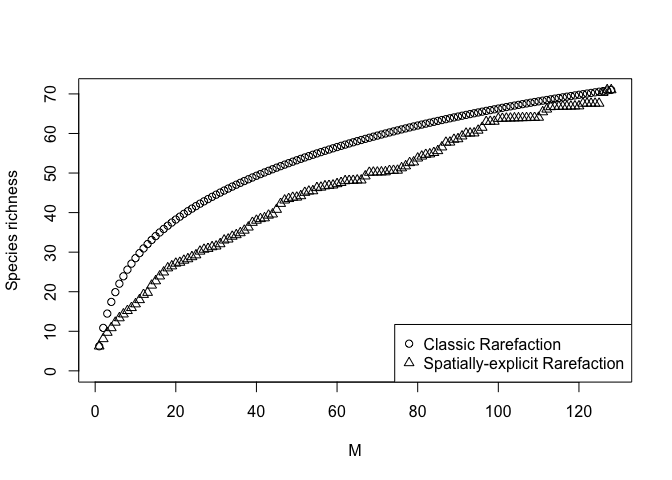## 3. Rarefaction of alpha diversity indices

In this example, the Shannon diversity index is rarefied over the M sampling units available for the duneFVG dataset. The argument fun_div allows the user to define any index of diversity of choice, and the function rare_alpha can be used to compare the spatial and non spatial-explicit rarefaction of the selected index. In the following example, two rarefaction curves (spatially and non-spatially explicit, respectively) are thus directly compared calling the function speciesdiv() of the adiv package (Pavoine 2020).

Firstly, a list containing the arguments for the function speciesdiv() is created such as the function rare_alpha() can exploit the function speciesdiv() loading the arguments.

a<-list(NA,'Shannon')
names(a)<-c('comm','method')

Then, spatially and non-spatially Shannon values are calculated as a function of the sampling effort.

rare_shannon<-rare_alpha(duneFVG$total,method="fun_div",random=999,fun_div='speciesdiv',args=a,mean=TRUE) rare_shannon_sp<-rare_alpha(duneFVG$total,dist_sp,method="fun_div",random=999,fun_div='speciesdiv',args=a,mean=TRUE,spatial=TRUE)
plot(rare_shannon[,1],ylab="Shannon index",xlab="Number of sampling units",type="l", ylim=range(rare_shannon,na.rm=TRUE))
lines(rare_shannon[,2],lty=2)
lines(rare_shannon[,3],lty=2)
lines(rare_shannon_sp[,1],col=4)
lines(rare_shannon_sp[,2],lty=2,col=4)
lines(rare_shannon_sp[,3],lty=2,col=4)
legend("bottomright",legend=c("Non spatially-explicit Rarefaction","Spatially-explicit Rarefaction"),lty=1,col=c(1,4))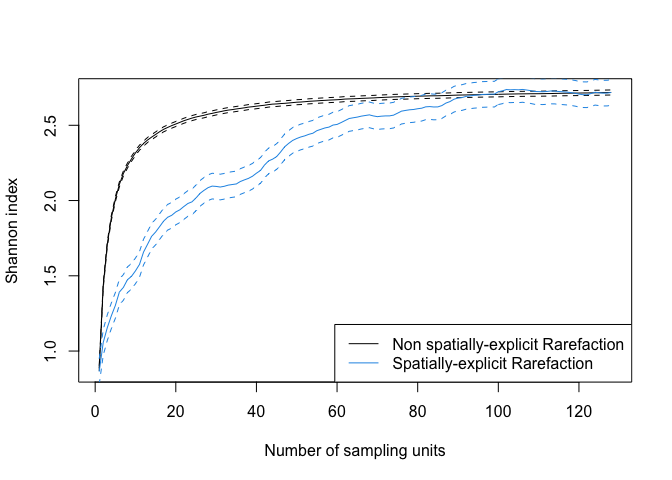The above figure shows the comparison of spatially and non-spatially explicit Shannon values for a given sampling effort along with 95% confidence intervals (dashed lines). When accounting for spatial structure of the data, the expected diversity increased less steeply than its non spatially-explicit counterpart resulting in lower estimates of species diversity.

## 4. Rarefaction of beta diversity

Spatially-explicit or gradient-oriented (directional) turnover curves as a function of sampling effort have been firstly defined by Ricotta et al. (2019). The methodology to construct the directional curve relies on the standard procedure in which adjacent plots are combined step by step using the specified distance among plots as a constraining factor. In the simplest case, given a set of N plots, for each plot, the first, second, ..., k-th nearest neighbor are determined and a directional beta diversity curve is constructed using the resulting sequence of plots. This procedure is repeated for all plots, generating N directional curves from which a mean spatially explicit beta diversity curve is calculated. The resulting curve is thus an intermediate solution between a non-directional beta diversity curve and a pure directional curve in which all plots are ordered along a single spatial or environmental gradient. directionalSAC can be used to calculate directional, normalized directional, non-directional, normalized non-directional beta diversity as a function of sampling effort. A normalized measure of autocorrelation for directional beta diversity calculated as the normalized difference between directional and non-directional beta is also available.

An example of the directional rarefaction of beta diversity is here provided using the mite dataset available in the vegan package (Oksanen et al. 2020). The same example is discussed in Appendix 1 in Ricotta et al. (2019).

data(mite)
data(mite.env)
comm_matrix<-mite 

To calculate and compare directional and non directional beta diversity along the environmental gradient defined according to the substrate density (g/L), the directionalSAC function can be used as follows:

beta_directional<-directionalSAC(comm_matrix,mite.env$SubsDens) Finally, directional and non-directional beta curves can be visually compared. plot(1:70,beta_directional$Beta_M,xlab="M",ylab="Beta diversity",ylim=range(c(beta_directional$Beta_M_dir,beta_directional$Beta_M)))
points(1:70,beta_directional$Beta_M_dir,pch=2) legend("bottomright",legend=c("Non-directional beta","Directional beta"),pch=1:2)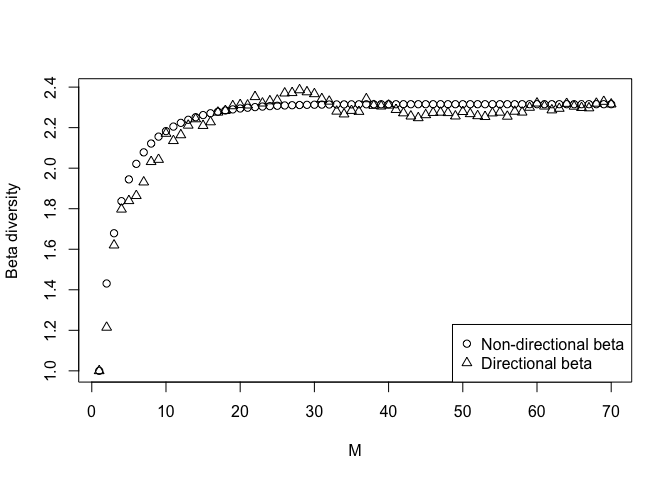## 5. Spatially-explicit phylogenetic rarefaction curve Rarefy presents for the first time the possibility to calculate spatially-explicit or gradient-based functional and phylogenetic rarefaction curves. We provide hereafter an example based on data available in the package (for functional rarefaction) and data available in other packages (phylogenetic rarefaction). Here, we present an example of a spatially-explicit phylogenetic rarefaction curve. The function rare_phylo() for the calculation of phylogenetic rarefaction curves have been used to calculate the Faith index. Data from the package phyloregion (Daru et al. 2020) have been used. First, the africa data are loaded from the package phyloregion: this list contains a sparse community composition matrix of each woody plant species presences/absences within 50 × 50 km grid cells in South Africa, an object of class SpatialPolygonsDataFrame containing the grid cells covering the geographic extent of the study area, an object of class phylo describing the phylogeny of 1400 species, an object of class dist that is a distance matrix of phylogenetic beta diversity between all grid cells at the 50 × 50 km scale and a data.frame of IUCN conservation status of each woody species. data(africa) A distance matrix between plots is extracted from the object polys of class SpatialPolygonsDataFrame. #data are in wgs84, reference system was changed to a projected one (LAEA) Poli_LAEA<-spTransform(africa$polys,CRSobj='+proj=laea +lat_0=52 +lon_0=10 +x_0=4321000 +y_0=3210000 +ellps=GRS80 +units=m +no_defs')
# Warning in showSRID(uprojargs, format = "PROJ", multiline = "NO", prefer_proj
# = prefer_proj): Discarded datum Unknown based on GRS80 ellipsoid in Proj4
# definition
# Warning in spTransform(xSP, CRSobj, ...): NULL source CRS comment, falling back
# to PROJ string
# Warning in wkt(obj): CRS object has no comment
#we need to fix rownames in order to make them comparable to Poli_LAEA
rownames(africa$comm)<-sapply(rownames(africa$comm),function(x){gsub(pattern = "v",replacement = "", x)})
dista<-dist(coordinates(Poli_LAEA[1:50,]))

Then, the phylogenetic spatially and non spatially-explicit rarefaction curves using Faith index are calculated for a subset of the dataset.

raref<-ser_phylo(as.matrix(africa$comm[1:50,colSums(africa$comm)>0]),africa$phylo,dista,comparison=TRUE)  plot(raref[,1],ylab="Faith Index",xlab="Number of sampling units",type="l",ylim=range(raref,na.rm=TRUE)) lines(raref[,2],lty=2) lines(raref[,3],lty=2) lines(raref[,4],col=2) lines(raref[,5],lty=2,col=2) lines(raref[,6],lty=2,col=2) legend("bottomright",legend=c("spatially-explicit phylogenetic rarefaction","classic phylogenetic rarefaction"),lty=1,col=1:2)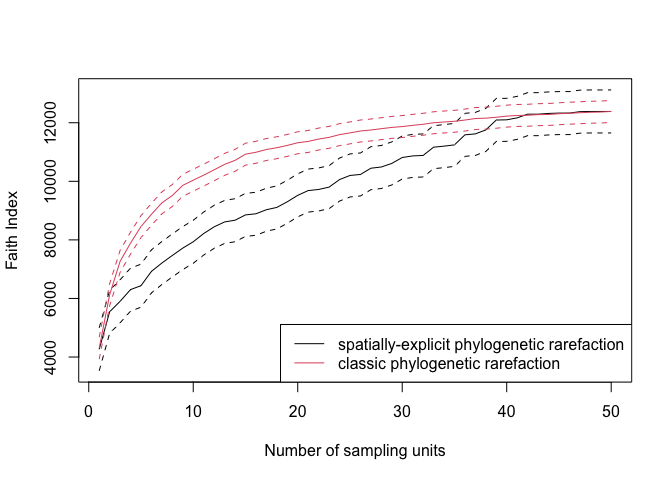In the figure figure, the phylogenetic rarefaction curves calculated usign the Faith index are compared. ## 6. Null models in rarefaction practice When communities are characterized by large difference in terms of species richness, it may be necessary to standardize rarefaction curves by accounting for this aspect. Null model based on re-sampling of the community can help to overcome the task. In the following example, discussed in Tordoni et al. (2019), we would like to compare functional rarefaction curves between native and alien species (62 and 9, respectively) sampled in the duneFVG study. In order to exclude that the expected lower functional diversity in alien species was merely driven by the imbalance in species number between alien and natives species, we built the rao_permuted function to test the expected functional rarefaction curve by means of species re-sampling and null model simulations. In brief, the function works in three stages: 1) from the total set of S species (62 native species in the example) sampled in M sampling units (128 plots in duneFVG), a defined number of s species (with s<S, 9 species in the example, corresponding to the alien species richness) is randomly selected; the s number of species is derived from a reference community (alien species in our example) passed to the function via the comm_str argument; 2) a functional dissimilarity matrix for the set of the s randomly selected species is calculated using their associated functional traits and then the rarefied Rao Q index is calculated for the set of M sampling units considering abundance of a reference plant community (the structure of the reference community passed to the function via the comm_str argument). 3) For each permutation, step 1 and 2 are repeated and the functional rarefaction curves are then averaged over the N permutations. The functional rarefaction curve thus obtained (that we can identify, in the example, as the average functional rarefaction curve of a community of 9 native species with the same structure of the reference alien community) can be compared to the functional rarefaction curve calculated for the community of reference, and the deviation from the two curves (when positive or negative) can be evaluated as an effect of environmental filtering, niche differentiation or biotic homogeneization, alternatively. In terms of code, the example can be run as follows: first data for alien and native species should be loaded and a functional pairwise distance matrix between native species using the Gower dissimilarity proposed by Pavoine et al. (2009) data(duneFVG.tr8) #species functional traits tr8_N<-duneFVG.tr8$traits.nat[,c(1,3,4)]
tr8_D<-data.frame(duneFVG.tr8$traits.nat[,2]) tr8_Q<-duneFVG.tr8$traits.nat[,5:15]
tr8dist<-dist.ktab(ktab.list.df(list(tr8_N,tr8_D,tr8_Q)),type=c('N','D','Q'))

The standardized functional rarefaction curve is then calculated using the pairwise functional distance of native species and the alien assemblage as reference community.

rareperm<-rao_permuted(duneFVG$alien,tr8dist) The functional rarefaction curves for the native and alien species are then calculated to be compared with the standardized functional rarefaction tr8n_N<-duneFVG.tr8$traits.nat[,c(1,3,4)]
tr8n_D<-data.frame(duneFVG.tr8$traits.nat[,2]) tr8n_Q<-duneFVG.tr8$traits.nat[,5:15]
tr8a_N<-duneFVG.tr8$traits.ali[,c(1,3,4)] tr8a_D<-data.frame(duneFVG.tr8$traits.ali[,2])
tr8a_Q<-duneFVG.tr8$traits.ali[,5:15] tr8ndist<-dist.ktab(ktab.list.df(list(tr8n_N,tr8n_D,tr8n_Q)),type=c('N','D','Q')) tr8adist<-dist.ktab(ktab.list.df(list(tr8a_N,tr8a_D,tr8a_Q)),type=c('N','D','Q')) raren<-rare_Rao(duneFVG$native,tr8ndist)
rarea<-rare_Rao(duneFVG\$alien,tr8adist)

And visually assessed to determine the degree of divergence

plot(raren[,1], ylab="Rao QE",xlab="Number of sampling units",type="l",ylim=range(raren))
lines(raren[,2],lty=2)
lines(raren[,3],lty=2)
lines(rareperm[,1],col=2)
lines(rareperm[,2],lty=2,col=2)
lines(rareperm[,3],lty=2,col=2)
lines(rarea[,1],col=4)
lines(rarea[,2],lty=2,col=4)
lines(rarea[,3],lty=2,col=4)
legend("bottomright", legend=c("Native species Functional Rarefaction","Standardized Functional Rarefaction","Alien species Functional Rarefaction"),lty=1,col=c(1,2,4))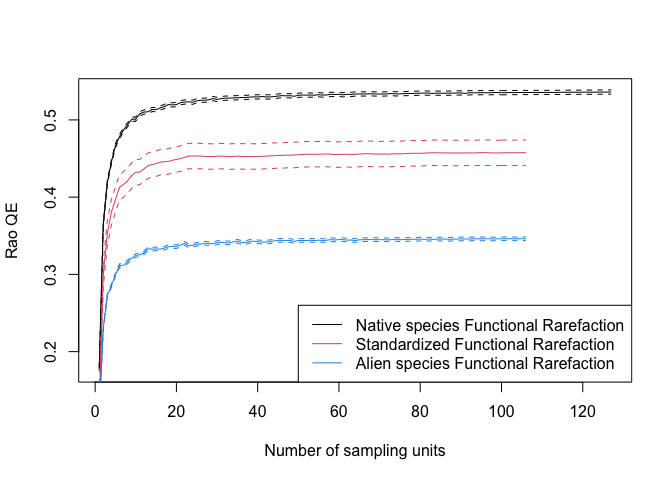## Cited Literature

Barker, G.M. (2002) Phylogenetic diversity: a quantitative framework for measurement of priority and achievement in biodiversity conservation. Biological Journal of the Linnean Society 76, 165-194.

Bray, J.R. & Curtis, J.T. (1957) An Ordination of the Upland Forest Communities of Southern Wisconsin. Ecological Monographs 27, 325-349.

Chao, A., Chiu, C.‐H., Villéger, S., Sun, I‐F., Thorn, S., Lin, Y.‐C., Chiang, J.‐M., Sherwin, W.B. (2019) An attribute‐diversity approach to functional diversity, functional beta diversity, and related (dis)similarity measures. Ecological Monographs 89(2), e01343.

Chiarucci, A., Bacaro, G., Rocchini, D., Ricotta, C., Palmer, M., & Scheiner S. (2009) Spatially constrained rarefaction: Incorporating the autocorrelated structure of biological communities into sample-based rarefaction. Community Ecology 10(2), 209-214.

Cody, M.L. (1975) Towards a theory of continental species diversities: bird distributions over Mediterranean habitat gradients. In: Cody M.L. & Diamond J.M. (eds) Ecology and Evolution of Communities (pp. 214--257). Harvard: Belknap Press.

Daròczy, Z. (1970) Generalized information functions. Inform. Control 16, 36-51.

Daru, B.H., Karunarathne, P. & Schliep, K. (2020) phyloregion: R package for biogeographic regionalization and macroecology. Methods Ecol Evol, 11, 1483-1491. .

Faith, DP. (1992) Conservation evaluation and phylogenetic diversity. Biol. Conserv. 61, 1--10.

Havrda, J., & Charvát, F. (1967) Quantification Method of Classification Processes. Concept of Structural-Entropy. Kybernetika 3, 30-35.

Hill, M.O. (1973) Diversity and Evenness: A Unifying Notation and Its Consequences. Ecology 54, 427-432.

Jaccard, P. (1912), The Distribution of the flora in the alpine zone. New Phytologist 11(2): 37--50.

Jaccard, P. (1901), Étude comparative de la distribution florale dans une portion des Alpes et des Jura. Bulletin de la Société vaudoise des sciences naturelles 37, 547--579.

Oksanen, J., Guillaume Blanchet, F., Friendly, M., Kindt, R., Legendre, P., McGlinn, D., ... Wagner H. (2020) vegan: Community Ecology Package. R package version 2.5-3.

Pavoine, S., Vallet, J., Dufour, A. B., Gachet, S., & Daniel, H. (2009) On the challenge of treating various types of variables: application for improving the measurement of functional diversity. Oikos, 118(3), 391-402.

Pavoine, S., & Ricotta, C. (2019) A simple translation from indices of species diversity to indices of phylogenetic diversity. Ecological Indicators 101, 552-561.

Pavoine, S. (2020) adiv: an R package to analyse biodiversity in ecology. Methods Ecol Evol, 11, 1106-1112.

Rao C.R. (1982) Diversity and dissimilarity coefficients: a unified approach. Theoretical Population Biology 21, 24-43.

Ricotta, C., Acosta, T.R.A., Bacaro, G., Carboni, M., Chiarucci, A., Rocchini, D., Pavoine, S. (2019) Rarefaction of beta diversity. Ecolological Indicators, 107, 105606.

Tordoni, E., Petruzzellis, F., Nardini, A., Savi, T., & Bacaro, G. (2019) Make it simpler: Alien species decrease functional diversity of coastal plant communities. J Veg Sci, 30, 498-- 509.

Tsallis, C. (1988) Possible generalization of Boltzamnn-Gibbs Statistics. J. Stat. Phys. 52, 479-487.

Whittaker, R.H. (1972) Evolution and measurement of species diversity. Taxon 21, 213-- 251.

Whittaker, R.H. (1960) Vegetation of the Siskiyou mountains, Oregon and California. Ecological Monographs 30, 279--338.